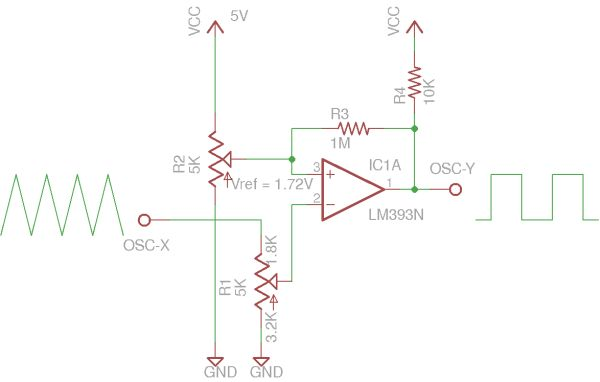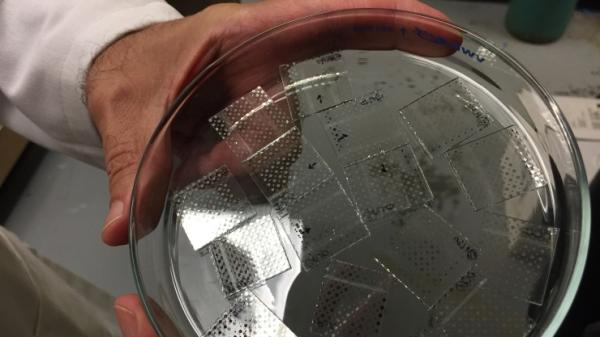Visualizing comparator and Op Amp hysteresis

Hysteresis can be added to a comparator circuit to improve its stability, especially when the input signal is noisy. In this post, we will examine the hysteresis characteristics of some common comparator and Op Amps using an oscilloscope.Perhaps the most intuitive way to visualize the hysteresis in a circuit is to plot the input signal (x axis) against the output signal (y axis). So, if we sweep the input voltage we should be able to see the characteristics of the transitioning of the output voltage due to hysteresis.

R1 (potentiometer) and R3 determine the trip voltage and hysteresis. Potentiometer R2 serves as a crude voltage reference and sets the reference voltage at the inverting input. R4 is a pull up resistor for comparators with open-collector outputs.

Here is a picture of the setup on breadboard. The potentiometer on the left (which corresponds to R1) sets the reference voltage at 1.72V. This value is used for all the tests conducted below. The actual voltage set for the reference is not very important however, it was mainly chosen for easy visualization and comparison purpose. The potentiometer (R1) connected to the negative input was adjusted to be 1.8K and 3.2K (from input to wiper, and wiper to ground). Again, the wiper position is fixed for all the tests.

Because a comparator’s hysteresis depends on many different factors such as the output voltage swing and input bias current, etc., you will see from the pictures below that even with the other settings unchanged (i.e. same resistor values), the hysteresis changes significantly depending on the comparator/op amps used. So to calculate hysteresis accurately, the characteristics of the comparator/op amp must be precisely known.

For more detail: Visualizing comparator and Op Amp hysteresis